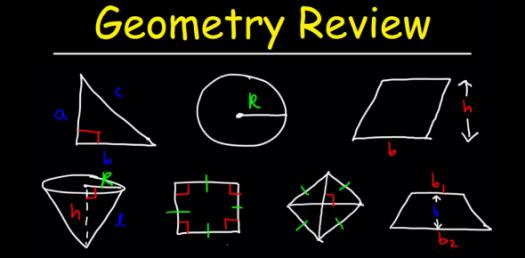# Geometry Practice Quiz: Triangles

25 Questions | Total Attempts: 84SettingsIsosceles Triangles, APRIL 6

Questions and Answers
• 1.
Find the value of x.
• 2.
Find the value of w.
• 3.
Find the value of x.
• 4.
Find the value of y.
• 5.
Find the value of z.
• 6.
Find the value of y.
• 7.
Find the value of x.
• 8.
Answer the question.
• A.

A

• B.

B

• C.

C

• D.

D

• E.

E

• 9.
Answer the question.
• A.

A

• B.

B

• C.

C

• D.

D

• E.

E

• 10.
Answer the question.
• A.

A

• B.

B

• C.

C

• D.

D

• E.

E

• 11.
Find the value of x.
• 12.
Find the value of y.
• 13.
Find the value of z.
• 14.
Find the value of y.
• 15.
Find the value of x.
• 16.
Find the value of x.
• 17.
Find the value of y.
• 18.
Find the value of y.
• 19.
Find the value of x.
• 20.
Find the value of y.
• 21.
Find the value of x.
Related Topics